Business Research Method Factor Analysis Factor Analysis Factor

• Slides: 52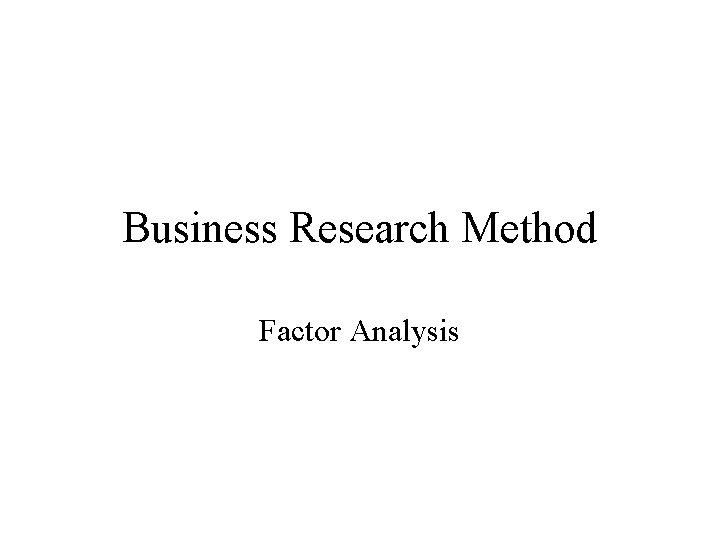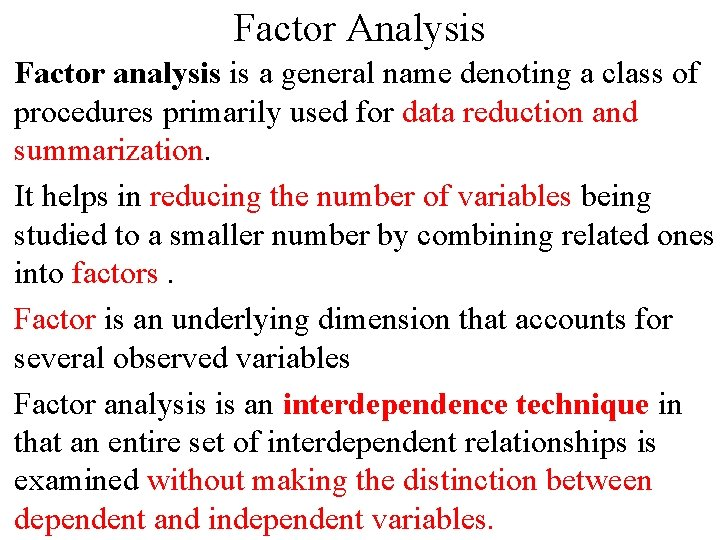Factor Analysis Factor analysis is a general name denoting a class of procedures primarily used for data reduction and summarization. It helps in reducing the number of variables being studied to a smaller number by combining related ones into factors. Factor is an underlying dimension that accounts for several observed variables Factor analysis is an interdependence technique in that an entire set of interdependent relationships is examined without making the distinction between dependent and independent variables.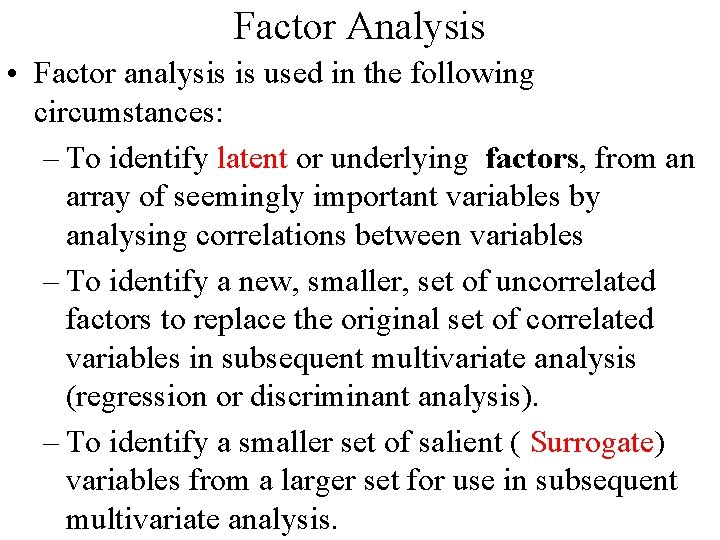Factor Analysis • Factor analysis is used in the following circumstances: – To identify latent or underlying factors, from an array of seemingly important variables by analysing correlations between variables – To identify a new, smaller, set of uncorrelated factors to replace the original set of correlated variables in subsequent multivariate analysis (regression or discriminant analysis). – To identify a smaller set of salient ( Surrogate) variables from a larger set for use in subsequent multivariate analysis.Factor Analysis • Evaluate credit card usage & behavior of customers. • Initial set of variables is large: Age, Gender, Marital status , Income, education, employment status, credit history& family background • Reduction of 9 variables in 3 factors : --Demographic Characteristic (Age, Gender, Marital status) --Socio-economic Status (Income, education, employment status ) --Background status (credit history& family background)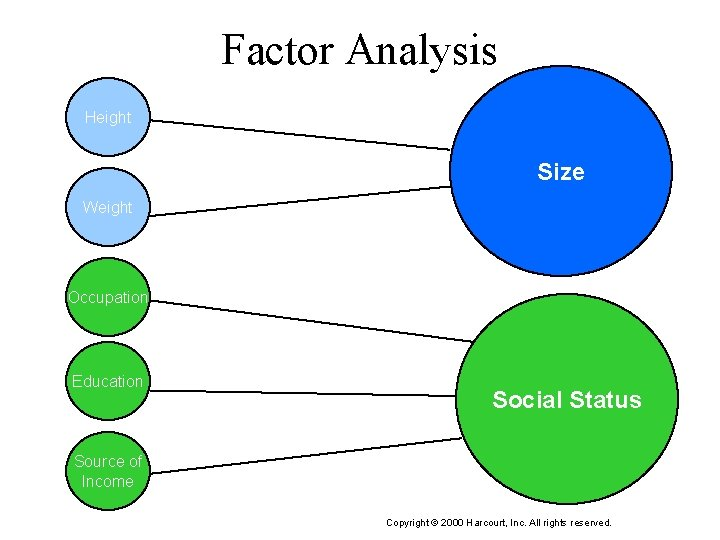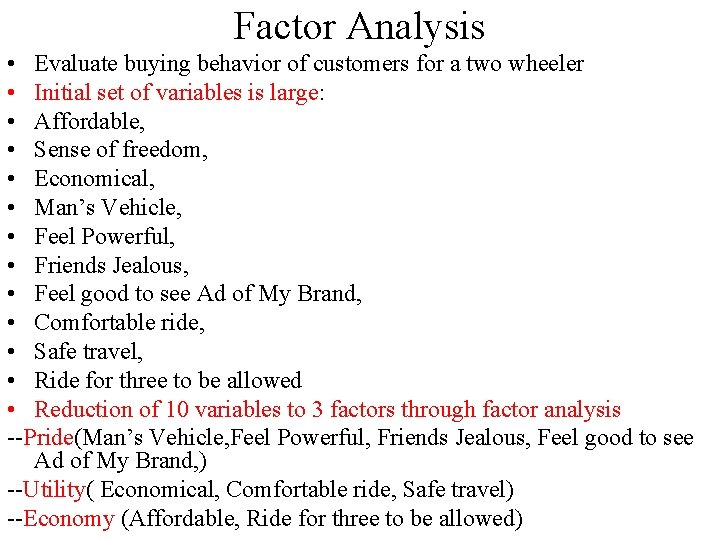Factor Analysis • Evaluate buying behavior of customers for a two wheeler • Initial set of variables is large: • Affordable, • Sense of freedom, • Economical, • Man’s Vehicle, • Feel Powerful, • Friends Jealous, • Feel good to see Ad of My Brand, • Comfortable ride, • Safe travel, • Ride for three to be allowed • Reduction of 10 variables to 3 factors through factor analysis --Pride(Man’s Vehicle, Feel Powerful, Friends Jealous, Feel good to see Ad of My Brand, ) --Utility( Economical, Comfortable ride, Safe travel) --Economy (Affordable, Ride for three to be allowed)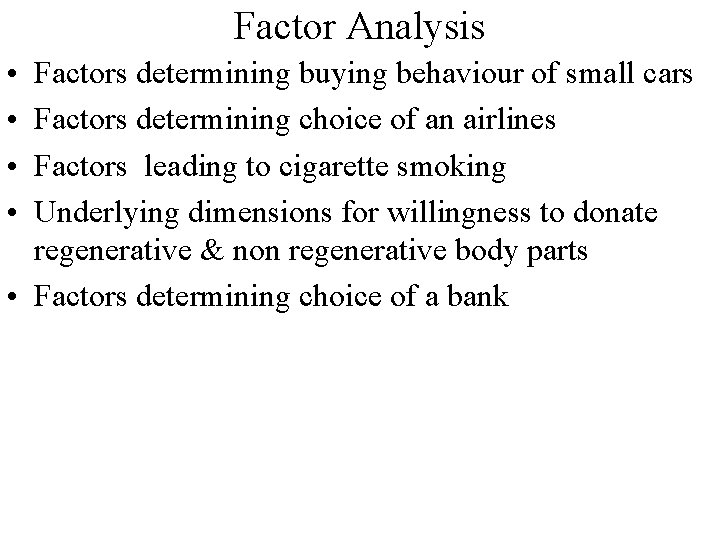Factor Analysis • • Factors determining buying behaviour of small cars Factors determining choice of an airlines Factors leading to cigarette smoking Underlying dimensions for willingness to donate regenerative & non regenerative body parts • Factors determining choice of a bankFactor Analysis Two Stages in Factor Analysis ----Factor Extraction ----Factor rotationFactor Extraction • • Determines Number of factors to be extracted Factors are linear combinations of original variables Maximum number of factors equals no. of variables Purpose is to reduce variables to fewer no. of factors Popular method is Principal Component Analysis. Based on the Concept of Eigen Value Higher the eigen value of the factor, higher is the amount of variance explained by the factor • Extract least number of factors to explain maximum varianceFactor Analysis-Extraction • Each original variable has Eigen value =1 due to standardization • Only factors with eigen value >= 1 are retained • Factors with eigen value < 1 are no better than a single variable • The number of factors extracted is determined so that cumulative % of variance extracted reaches a satisfactory level ( at least 60% )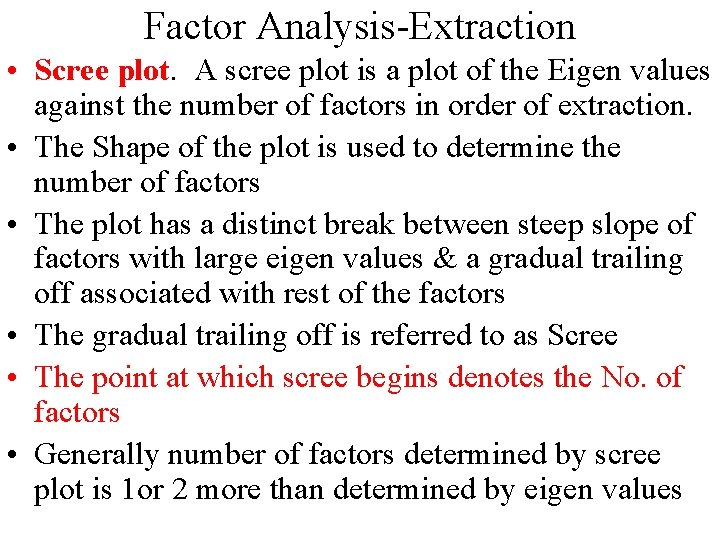Factor Analysis-Extraction • Scree plot. A scree plot is a plot of the Eigen values against the number of factors in order of extraction. • The Shape of the plot is used to determine the number of factors • The plot has a distinct break between steep slope of factors with large eigen values & a gradual trailing off associated with rest of the factors • The gradual trailing off is referred to as Scree • The point at which scree begins denotes the No. of factors • Generally number of factors determined by scree plot is 1 or 2 more than determined by eigen values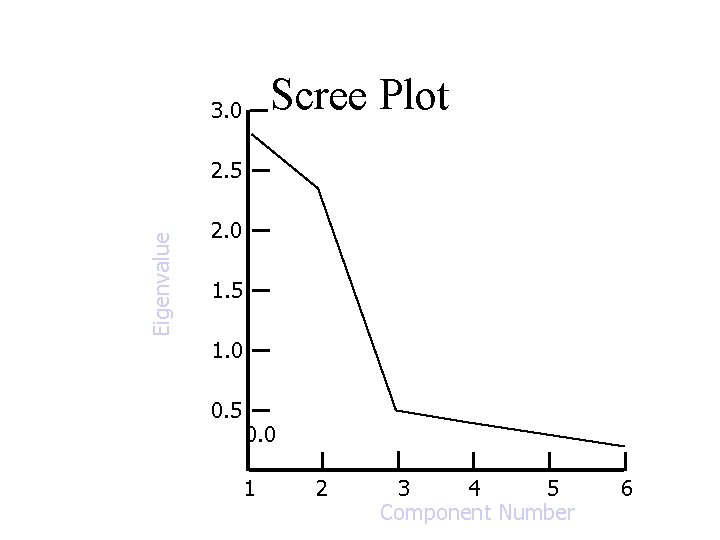Scree Plot 3. 0 Eigenvalue 2. 5 2. 0 1. 5 1. 0 0. 5 0. 0 1 2 3 4 5 Component Number 6Factor rotation • After extraction the next task is to interpret & name the factors • This is done by identifying which factors are associated with which original variables • The factor matrix is used for this purpose • The original factor matrix is unrotated & comes as output of stage I • The rotated factor matrix comes as output of stage II when we request the computer package to perform rotation & give us a rotated factor matrix • The popular method of rotation is Orthogonal(varimax )Factor rotation • Although the initial or unrotated factor matrix indicates the relationship between the factors and individual variables, it seldom results in factors that can be interpreted, because the factors are correlated with many variables. • Therefore, through rotation the factor matrix is transformed into a simpler one that is easier to interpret. • In rotating the factors, we would like each factor to have nonzero, or significant, loadings or coefficients for only some of the variables. • Rotation does not affect communalities& % of total variance explained. However % of variance accounted for by each factor does change • In factor rotation smallest loadings tend towards 0 & largest loadings tend towards 1. • The rotation is called orthogonal rotation if the axes are maintained at right angles.• • • Example To determine benefits consumer seeks from purchase of a toothpaste A sample of 30 respondents was interviewed Respondents were asked to indicate their degree of agreement with the following statements using a 7 point scale(1=Strongly agree, 7= Strongly disagree) V 1: Important to buy a toothpaste that prevents cavities V 2: Like a toothpaste that gives shiny teeth V 3: A toothpaste should strengthen your gums V 4: Prefer toothpaste that freshens breath V 5: Prevention of tooth decay is not an important benefit V 6: The most important consideration is attractive teeth Data obtained are given in the next slideConducting Factor AnalysisFormulate the Problem • The objectives of factor analysis should be identified. • The variables to be included in the factor analysis should be specified based on past research, theory, and judgment of the researcher. It is important that the variables be appropriately measured on an interval or ratio scale. • An appropriate sample size should be used. As a rough guideline, there should be at least four or five times as many observations (sample size) as there are variables.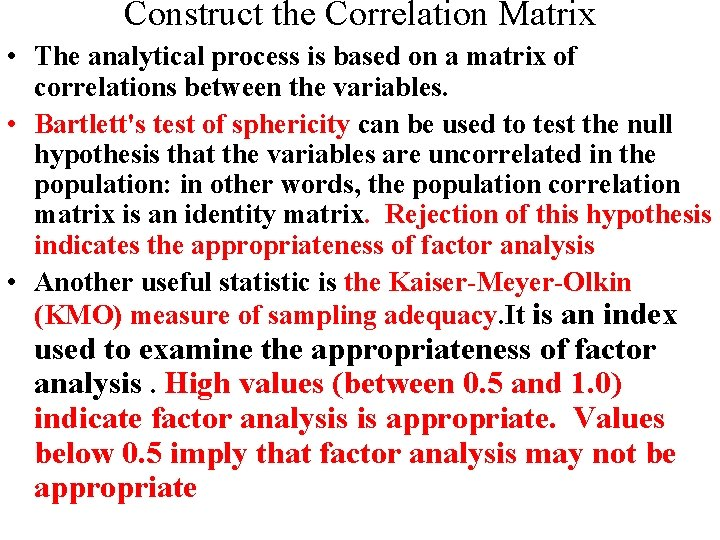Construct the Correlation Matrix • The analytical process is based on a matrix of correlations between the variables. • Bartlett's test of sphericity can be used to test the null hypothesis that the variables are uncorrelated in the population: in other words, the population correlation matrix is an identity matrix. Rejection of this hypothesis indicates the appropriateness of factor analysis • Another useful statistic is the Kaiser-Meyer-Olkin (KMO) measure of sampling adequacy. It is an index used to examine the appropriateness of factor analysis. High values (between 0. 5 and 1. 0) indicate factor analysis is appropriate. Values below 0. 5 imply that factor analysis may not be appropriate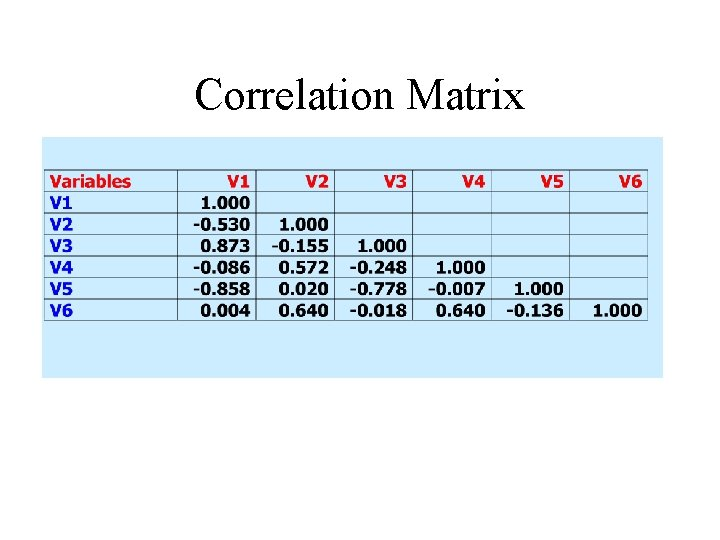Correlation Matrix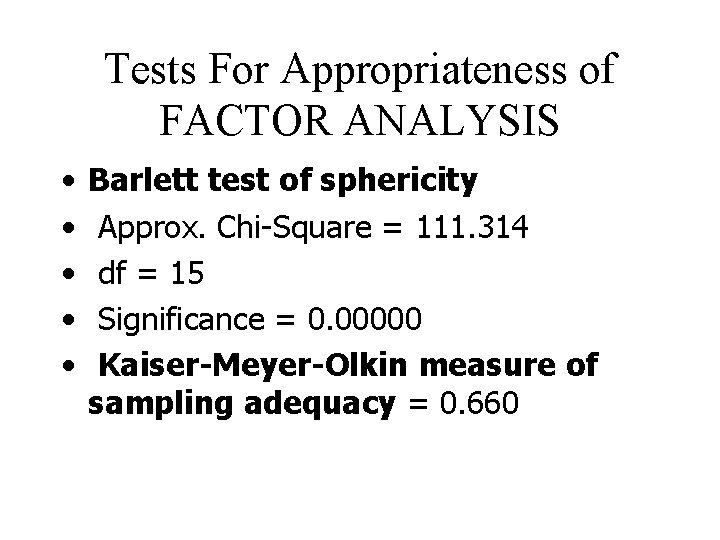Tests For Appropriateness of FACTOR ANALYSIS • • • Barlett test of sphericity Approx. Chi-Square = 111. 314 df = 15 Significance = 0. 00000 Kaiser-Meyer-Olkin measure of sampling adequacy = 0. 660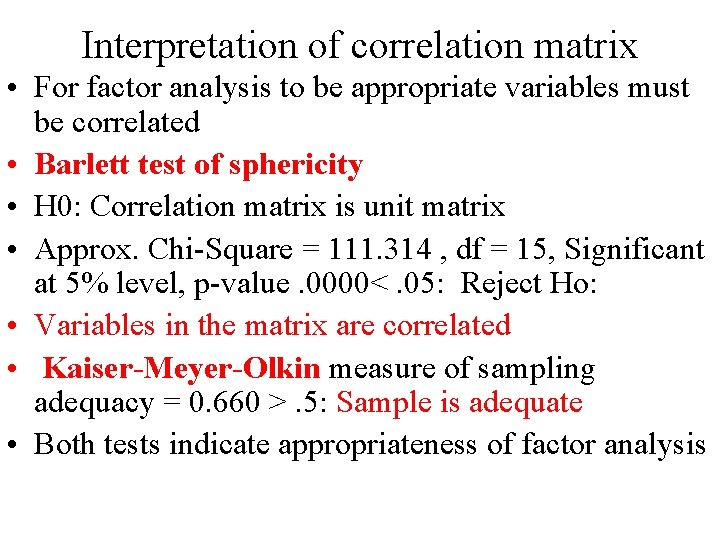Interpretation of correlation matrix • For factor analysis to be appropriate variables must be correlated • Barlett test of sphericity • H 0: Correlation matrix is unit matrix • Approx. Chi-Square = 111. 314 , df = 15, Significant at 5% level, p-value. 0000<. 05: Reject Ho: • Variables in the matrix are correlated • Kaiser-Meyer-Olkin measure of sampling adequacy = 0. 660 >. 5: Sample is adequate • Both tests indicate appropriateness of factor analysis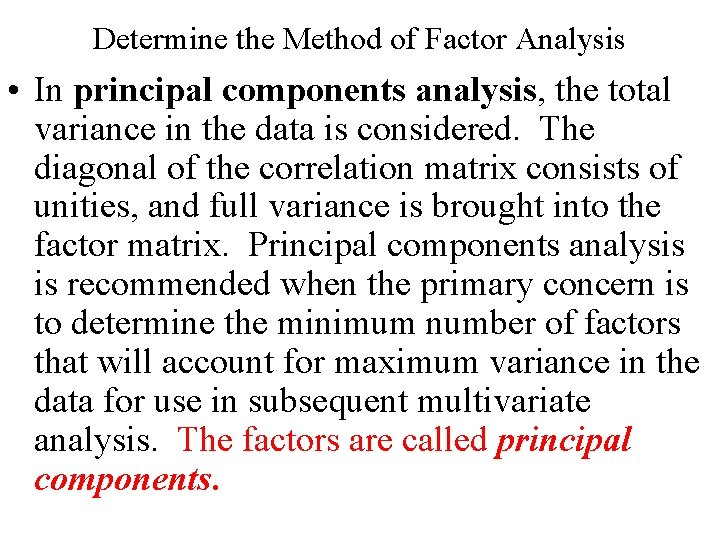Determine the Method of Factor Analysis • In principal components analysis, the total variance in the data is considered. The diagonal of the correlation matrix consists of unities, and full variance is brought into the factor matrix. Principal components analysis is recommended when the primary concern is to determine the minimum number of factors that will account for maximum variance in the data for use in subsequent multivariate analysis. The factors are called principal components.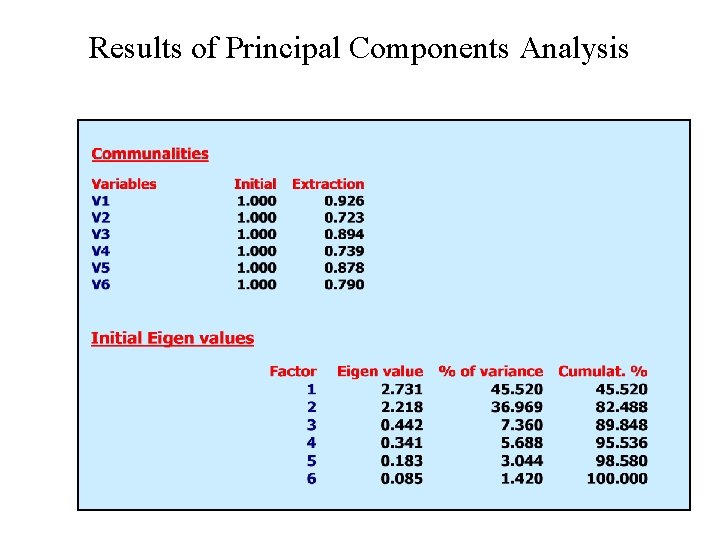Results of Principal Components Analysis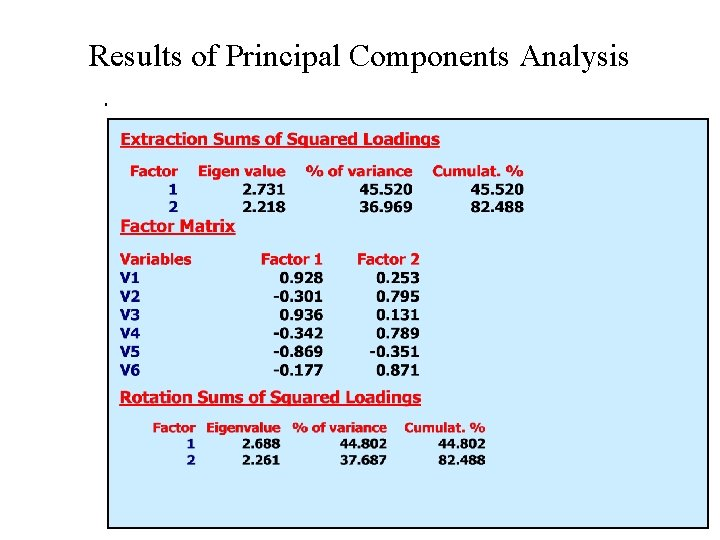Results of Principal Components Analysis.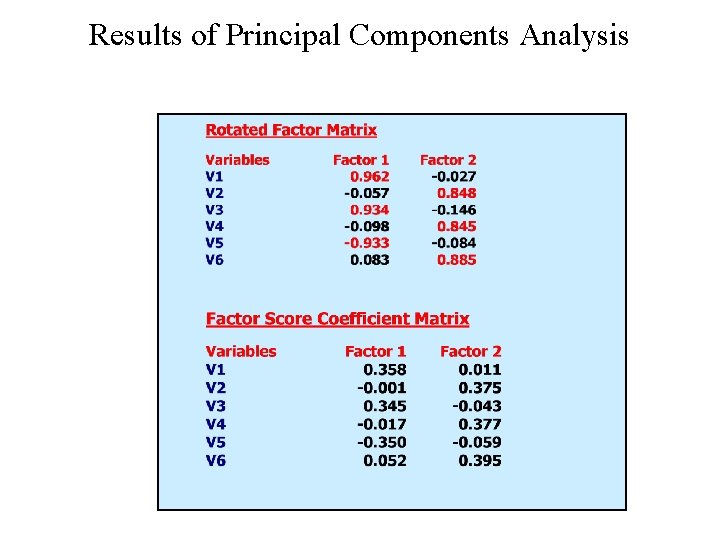Results of Principal Components Analysis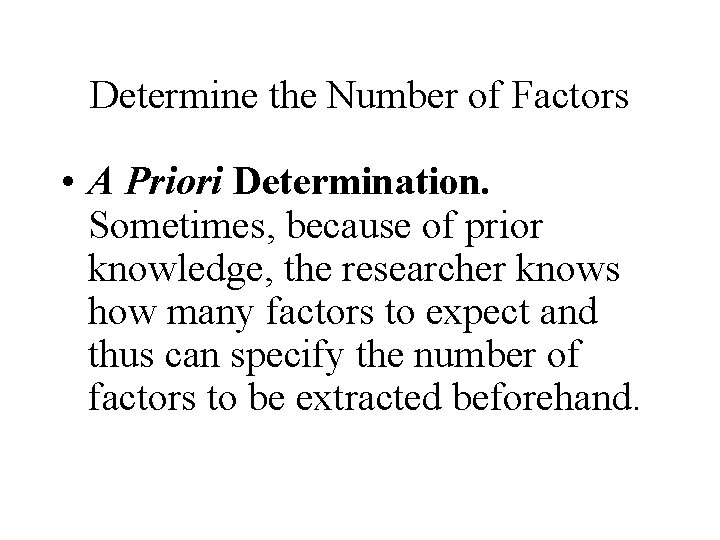Determine the Number of Factors • A Priori Determination. Sometimes, because of prior knowledge, the researcher knows how many factors to expect and thus can specify the number of factors to be extracted beforehand.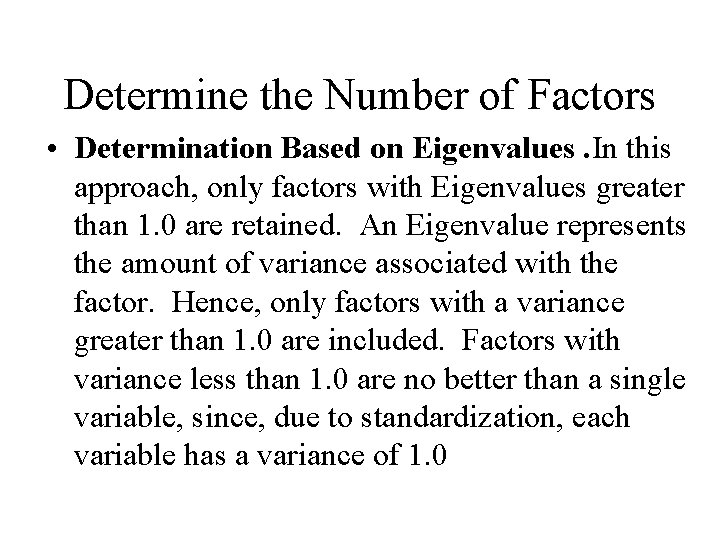Determine the Number of Factors • Determination Based on Eigenvalues. In this approach, only factors with Eigenvalues greater than 1. 0 are retained. An Eigenvalue represents the amount of variance associated with the factor. Hence, only factors with a variance greater than 1. 0 are included. Factors with variance less than 1. 0 are no better than a single variable, since, due to standardization, each variable has a variance of 1. 0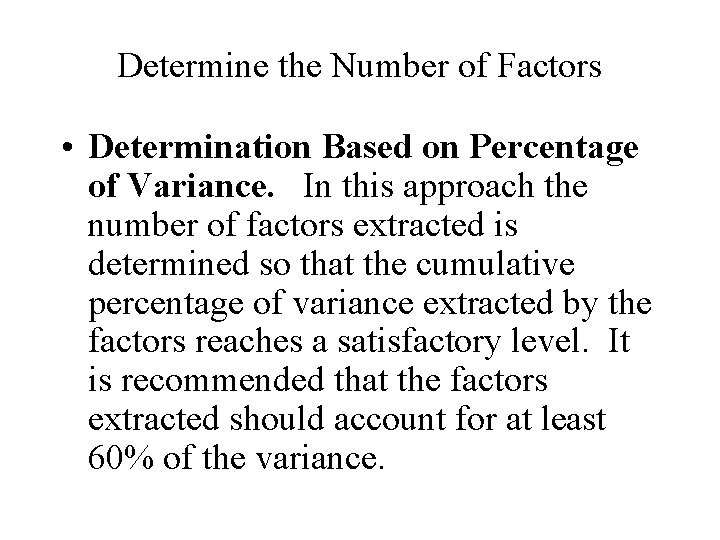Determine the Number of Factors • Determination Based on Percentage of Variance. In this approach the number of factors extracted is determined so that the cumulative percentage of variance extracted by the factors reaches a satisfactory level. It is recommended that the factors extracted should account for at least 60% of the variance.Determine the Number of Factors • Determination Based on Scree Plot. A scree plot is a plot of the Eigenvalues against the number of factors in order of extraction. Experimental evidence indicates that the point at which the scree begins denotes the true number of factors. Generally, the number of factors determined by a scree plot will be one or a few more than that determined by the Eigenvalue criterion.Fig 19. 2 Scree Plot 3. 0 Eigenvalue 2. 5 2. 0 1. 5 1. 0 0. 5 0. 0 1 2 3 4 5 Component Number 6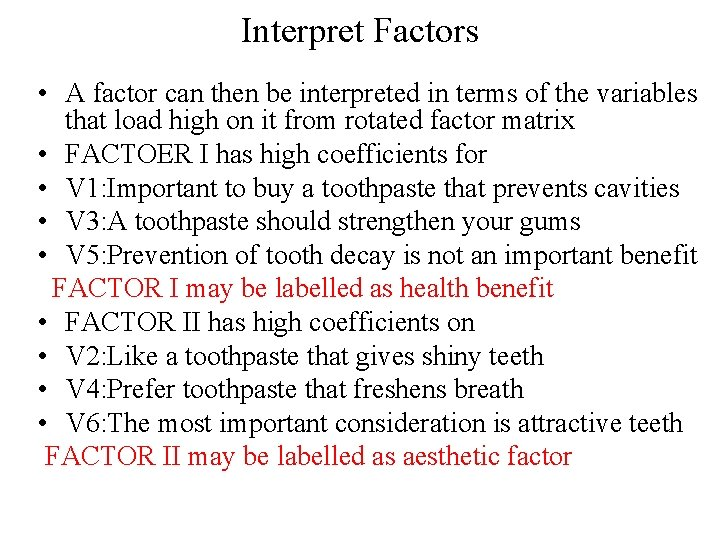Interpret Factors • A factor can then be interpreted in terms of the variables that load high on it from rotated factor matrix • FACTOER I has high coefficients for • V 1: Important to buy a toothpaste that prevents cavities • V 3: A toothpaste should strengthen your gums • V 5: Prevention of tooth decay is not an important benefit FACTOR I may be labelled as health benefit • FACTOR II has high coefficients on • V 2: Like a toothpaste that gives shiny teeth • V 4: Prefer toothpaste that freshens breath • V 6: The most important consideration is attractive teeth FACTOR II may be labelled as aesthetic factorFactor Loading Plot • Another useful aid in interpretation is to plot the variables, using the factor loadings as coordinates. Variables at the end of an axis are those that have high loadings on only that factor, and hence describe the factor.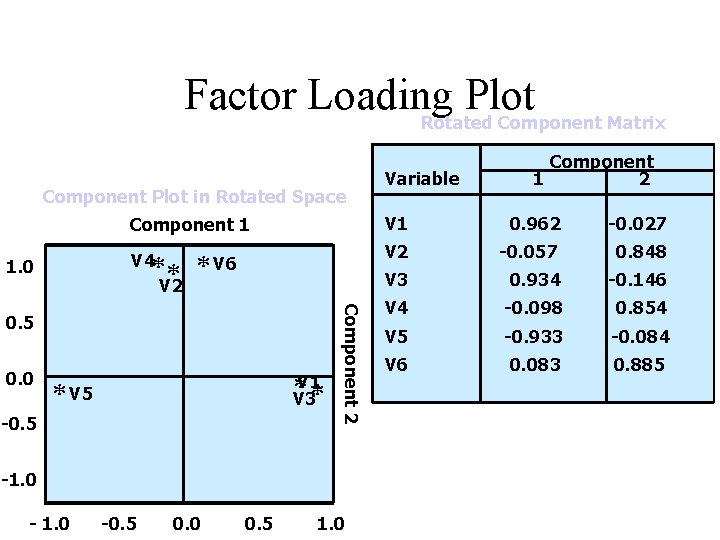Factor Loading Plot Rotated Component Matrix Component Plot in Rotated Space Component 1 ** * V 4 1. 0 V 6 V 2 0. 0 V 1 * V 3* * V 5 -0. 5 Component 2 0. 5 -1. 0 -0. 5 0. 0 0. 5 1. 0 Variable Component 1 2 V 1 0. 962 -0. 027 V 2 -0. 057 0. 848 V 3 0. 934 -0. 146 V 4 -0. 098 0. 854 V 5 -0. 933 -0. 084 V 6 0. 083 0. 885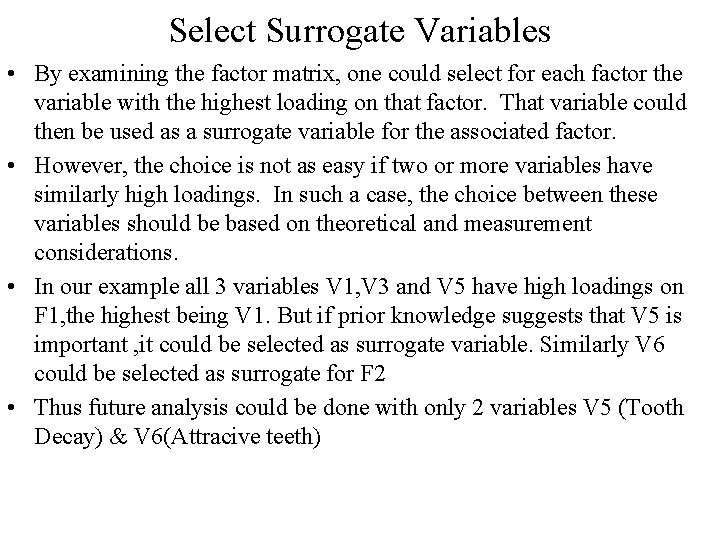Select Surrogate Variables • By examining the factor matrix, one could select for each factor the variable with the highest loading on that factor. That variable could then be used as a surrogate variable for the associated factor. • However, the choice is not as easy if two or more variables have similarly high loadings. In such a case, the choice between these variables should be based on theoretical and measurement considerations. • In our example all 3 variables V 1, V 3 and V 5 have high loadings on F 1, the highest being V 1. But if prior knowledge suggests that V 5 is important , it could be selected as surrogate variable. Similarly V 6 could be selected as surrogate for F 2 • Thus future analysis could be done with only 2 variables V 5 (Tooth Decay) & V 6(Attracive teeth)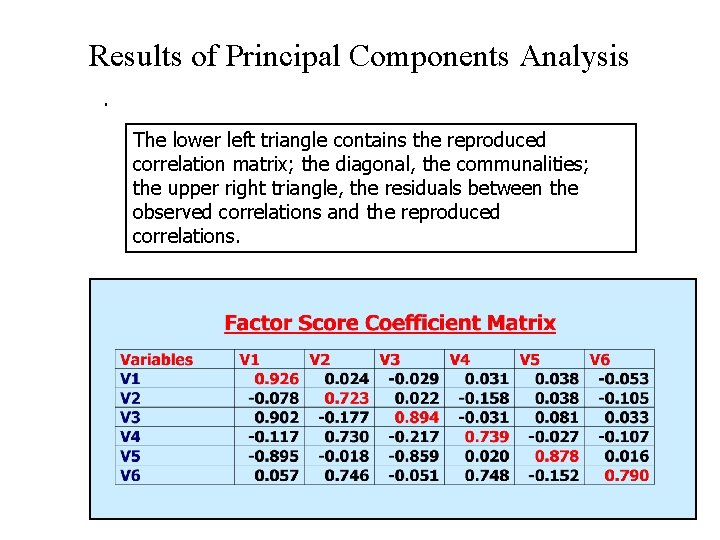Results of Principal Components Analysis. The lower left triangle contains the reproduced correlation matrix; the diagonal, the communalities; the upper right triangle, the residuals between the observed correlations and the reproduced correlations.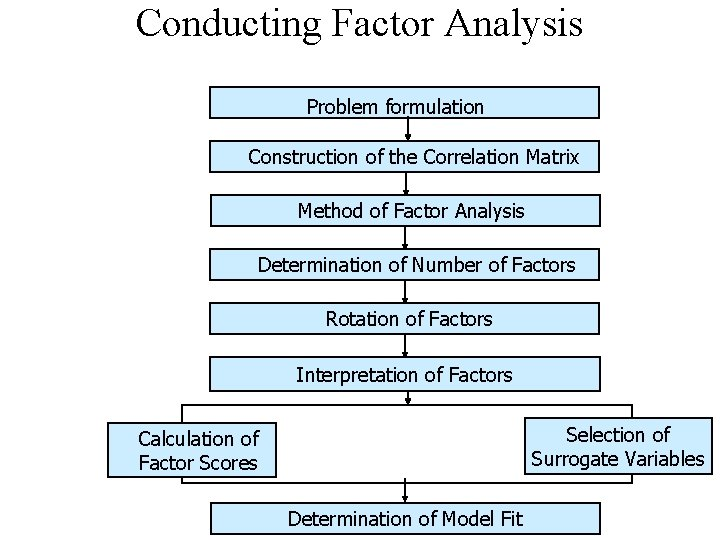Conducting Factor Analysis Problem formulation Construction of the Correlation Matrix Method of Factor Analysis Determination of Number of Factors Rotation of Factors Interpretation of Factors Selection of Surrogate Variables Calculation of Factor Scores Determination of Model FitFactor Analysis Model The factors can be expressed as linear combinations of the observed variables. Fi = Wi 1 X 1 + Wi 2 X 2 + Wi 3 X 3 +. . . + Wik. Xk where Fi = estimate of i th factor Wi = weight or factor score coefficient k = number of variablesFactor Analysis Model • It is possible to select weights or factor score coefficients so that the first factor explains the largest portion of the total variance. • Then a second set of weights can be selected, so that the second factor accounts for most of the residual variance, subject to being uncorrelated with the first factor. • This same principle could be applied to selecting additional weights for the additional factors.Statistics Associated with Factor Analysis • Bartlett's test of sphericity is a test statistic used to examine the null hypothesis that the variables are uncorrelated in the population. In other words, the population correlation matrix is an identity matrix; each variable correlates perfectly with itself (r = 1) but has no correlation with the other variables (r = 0) A large value of test statistics favors rejection of null hypothesis & factor analysis is meaningful. • Correlation matrix. A correlation matrix is a lower triangle matrix showing the simple correlations, r, between all possible pairs of variables included in the analysis. The diagonal elements, which are all 1, are usually omitted.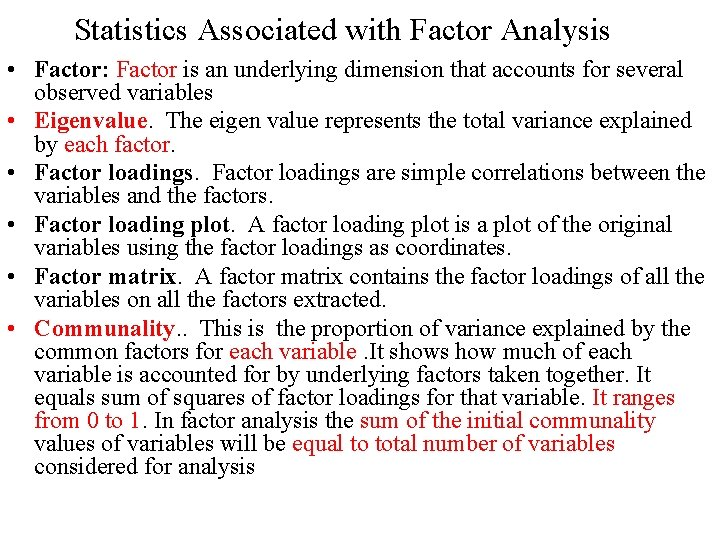Statistics Associated with Factor Analysis • Factor scores are composite scores estimated for each respondent on the derived factors. • Kaiser-Meyer-Olkin (KMO) measure of sampling adequacy. The Kaiser-Meyer-Olkin (KMO) measure of sampling adequacy is an index used to examine the appropriateness of factor analysis. High values (between 0. 5 and 1. 0) indicate factor analysis is appropriate. Values below 0. 5 imply that factor analysis may not be appropriate. • Percentage of variance. The percentage of the total variance attributed to each factor. • Residuals are the differences between the observed correlations, as given in the input correlation matrix, and the reproduced correlations, as estimated from the factor matrix. • Scree plot. A scree plot is a plot of the Eigenvalues against the number of factors in order of extraction.Appendix •Determine the Number of Factors • A Priori Determination. Sometimes, because of prior knowledge, the researcher knows how many factors to expect and thus can specify the number of factors to be extracted beforehand. • Determination Based on Eigenvalues. In this approach, only factors with Eigenvalues greater than 1. 0 are retained. An Eigenvalue represents the amount of variance associated with the factor. Hence, only factors with a variance greater than 1. 0 are included. Factors with variance less than 1. 0 are no better than a single variable, since, due to standardization, each variable has a variance of 1. 0. If the number of variables is less than 20, this approach will result in a conservative number of factors.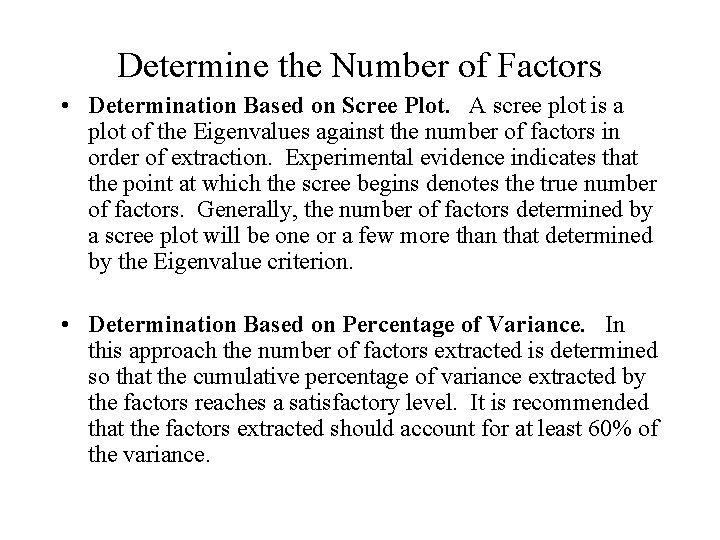Determine the Number of Factors • Determination Based on Scree Plot. A scree plot is a plot of the Eigenvalues against the number of factors in order of extraction. Experimental evidence indicates that the point at which the scree begins denotes the true number of factors. Generally, the number of factors determined by a scree plot will be one or a few more than that determined by the Eigenvalue criterion. • Determination Based on Percentage of Variance. In this approach the number of factors extracted is determined so that the cumulative percentage of variance extracted by the factors reaches a satisfactory level. It is recommended that the factors extracted should account for at least 60% of the variance.Fig 19. 2 Scree Plot 3. 0 Eigenvalue 2. 5 2. 0 1. 5 1. 0 0. 5 0. 0 1 2 3 4 5 Component Number 6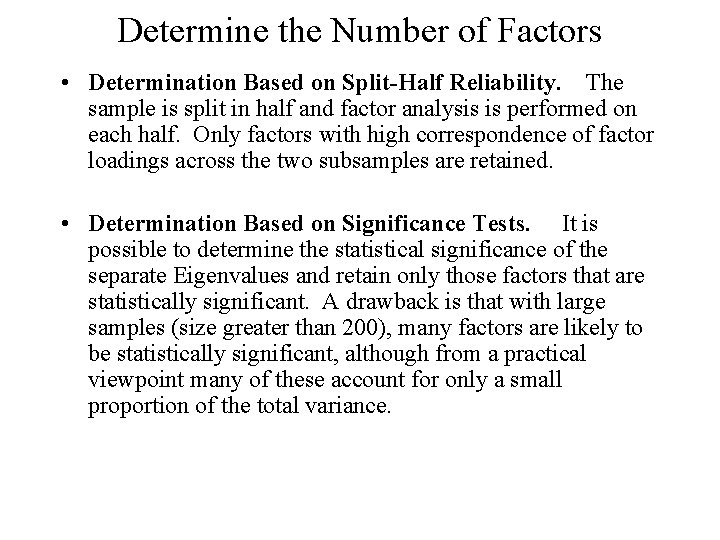Determine the Number of Factors • Determination Based on Split-Half Reliability. The sample is split in half and factor analysis is performed on each half. Only factors with high correspondence of factor loadings across the two subsamples are retained. • Determination Based on Significance Tests. It is possible to determine the statistical significance of the separate Eigenvalues and retain only those factors that are statistically significant. A drawback is that with large samples (size greater than 200), many factors are likely to be statistically significant, although from a practical viewpoint many of these account for only a small proportion of the total variance.Rotate Factors • Although the initial or unrotated factor matrix indicates the relationship between the factors and individual variables, it seldom results in factors that can be interpreted, because the factors are correlated with many variables. Therefore, through rotation the factor matrix is transformed into a simpler one that is easier to interpret. • In rotating the factors, we would like each factor to have nonzero, or significant, loadings or coefficients for only some of the variables. • The rotation is called orthogonal rotation if the axes are maintained at right angles.Rotate Factors • The most commonly used method for rotation is the varimax procedure. This is an orthogonal method of rotation that minimizes the number of variables with high loadings on a factor, thereby enhancing the interpretability of the factors. Orthogonal rotation results in factors that are uncorrelated. • The rotation is called oblique rotation when the axes are not maintained at right angles, and the factors are correlated. Sometimes, allowing for correlations among factors can simplify the factor pattern matrix. Oblique rotation should be used when factors in the population are likely to be strongly correlated.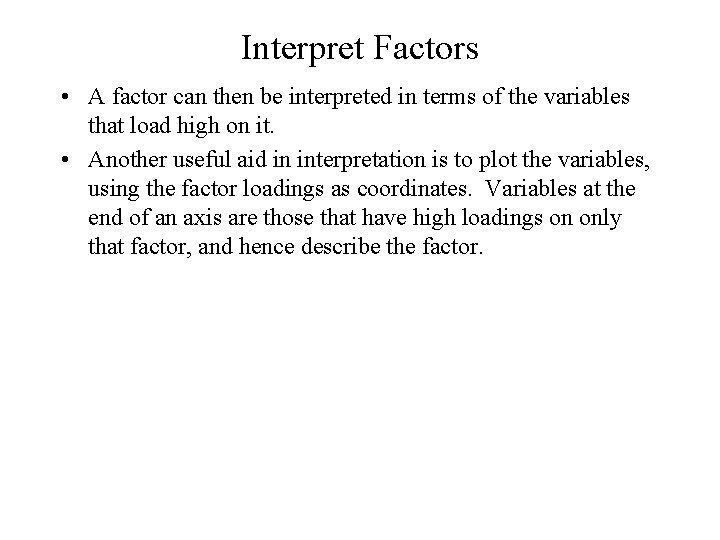Interpret Factors • A factor can then be interpreted in terms of the variables that load high on it. • Another useful aid in interpretation is to plot the variables, using the factor loadings as coordinates. Variables at the end of an axis are those that have high loadings on only that factor, and hence describe the factor.Select Surrogate Variables • By examining the factor matrix, one could select for each factor the variable with the highest loading on that factor. That variable could then be used as a surrogate variable for the associated factor. • However, the choice is not as easy if two or more variables have similarly high loadings. In such a case, the choice between these variables should be based on theoretical and measurement considerations.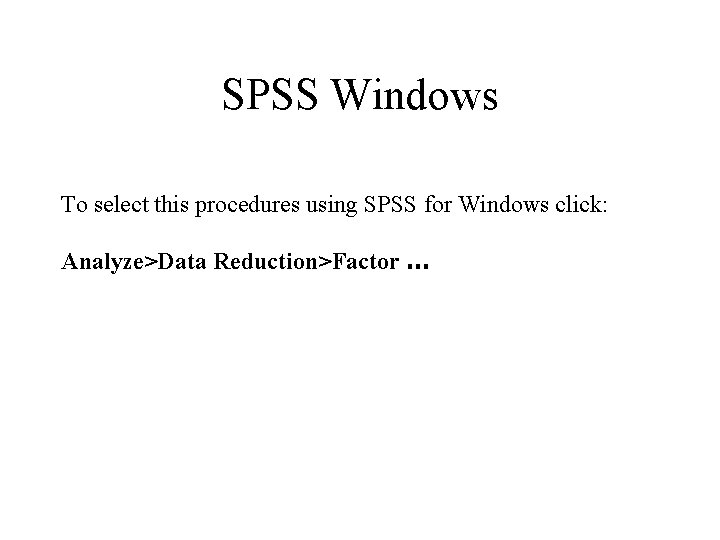SPSS Windows To select this procedures using SPSS for Windows click: Analyze>Data Reduction>Factor …